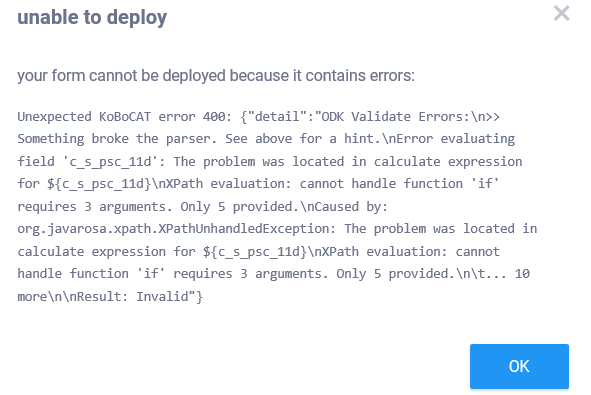Hi all,

I am trying to make a calculation of 17 questions together, to get a sum score,

I have looked at the very helpful article of Stephane Aloo: https://support.kobotoolbox.org/en/articles/1913851-advanced-use-of-calculate-questions

As Stephane describes in his part of ‘work around for caculations’, I would like to create dummy variables first as well, and then make a sumscore for all dummies together.

But the difference for us is that there is not just one response options that needs to be kept, but actually two.
The response options in our case are:

• PSC 0 never
- PSC 1 sometimes
- PSC 2 often
• PSC 66 Participant did not understand question
• PSC 88 (If voluntary mentioned) Don’t know
• PSC 99 (If voluntary mentioned) refused

So in this case we would like to sum up all 1’s and 2’s. So therefore I think we should have a nested ‘if’, like this:
if(\${c_s_psc_4}=2,2,if\${c_s_psc_4}=1,1,0)

Also for some items we use reverse scoring, so came up with:
if(\${c_s_psc_13}=0,2,if\${c_s_psc_13}=1,1,0)

But now it is giving an error code, see attached,for some of the items (only the one with double digits. So e.g.:
if(\${c_s_psc_11}=2,2,if\${c_s_psc_11}=1,1,0)

The first ones are not giving an error code in kobo:
if(\${c_s_psc_4}=2,2,if\${c_s_psc_4}=1,1,0)
if(\${c_s_psc_7}=2,2,if\${c_s_psc_7}=1,1,0)
if(\${c_s_psc_8}=2,2,if\${c_s_psc_8}=1,1,0)
if(\${c_s_psc_9}=2,2,if\${c_s_psc_9}=1,1,0)

How to get this calculation working?

best,

Frederik

Hi all,

solved. I forgot a parenthesis. So it should have been: if(\${c_s_psc_4}=2,2,if(\${c_s_psc_4}=1,1,0)) instead of if(\${c_s_psc_4}=2,2,if\${c_s_psc_4}=1,1,0) . So it should work now.

Best,

Frederik

2 Likes

Thank you for sharing your solution with the entire community! It should benefit users having similar issues.

FYI, you could also have a look at different `if-statement` that has been discussed in the forum here.

Have a great day!